# Long-Term GDP by US State, Second Quarter 2021

Nov 30, 2021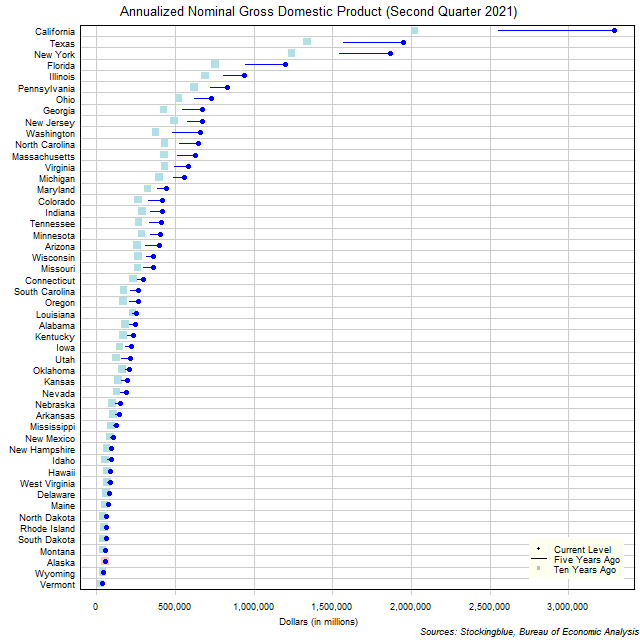The chart above shows the annualized nominal gross domestic product (GDP) in each US state as of the second quarter of 2021 in millions of dollars, the change from five years ago, and the GDP ten years prior.  California's economy grew more over the past five years than the size of 44 states' entire economies.

# EU and US GDP Growth Rate by Region, Second Quarter 2021

Nov 29, 2021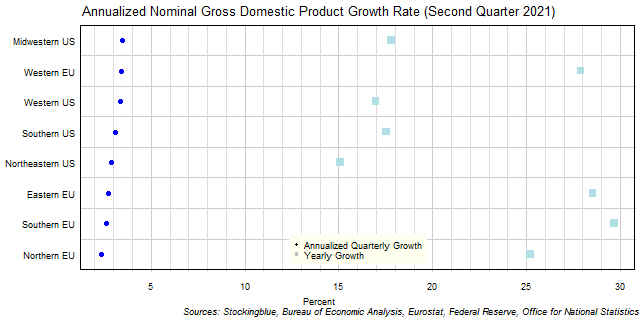The chart above shows the annualized nominal gross domestic product (GDP) quarterly growth rate in each EU and US region as of the second quarter of 2021 and the growth rate from one year prior.  Every single region's economy grew over the previous quarter.

# EU GDP Growth Rate by Region, Second Quarter 2021

Nov 26, 2021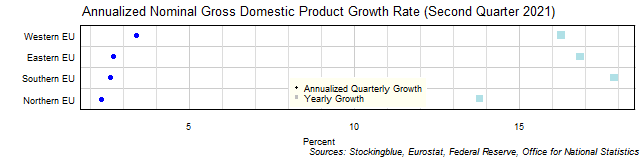The chart above shows the annualized nominal gross domestic product (GDP) quarterly growth rate in each EU region as of the second quarter of 2021 and the growth rate from one year prior.  All four regions grew over the previous quarter.

# US GDP Growth Rate by Region, Second Quarter 2021

Nov 25, 2021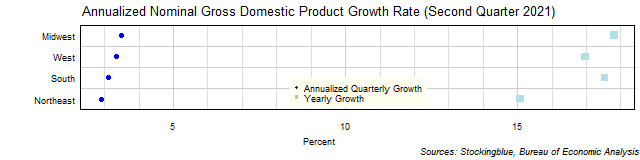The chart above shows the annualized nominal gross domestic product (GDP) quarterly growth rate in each US region as of the second quarter of 2021 and the growth rate from one year prior.  Every single region's economy grew over the previous quarter.

# EU and US GDP Growth Rate by State, Second Quarter 2021

Nov 24, 2021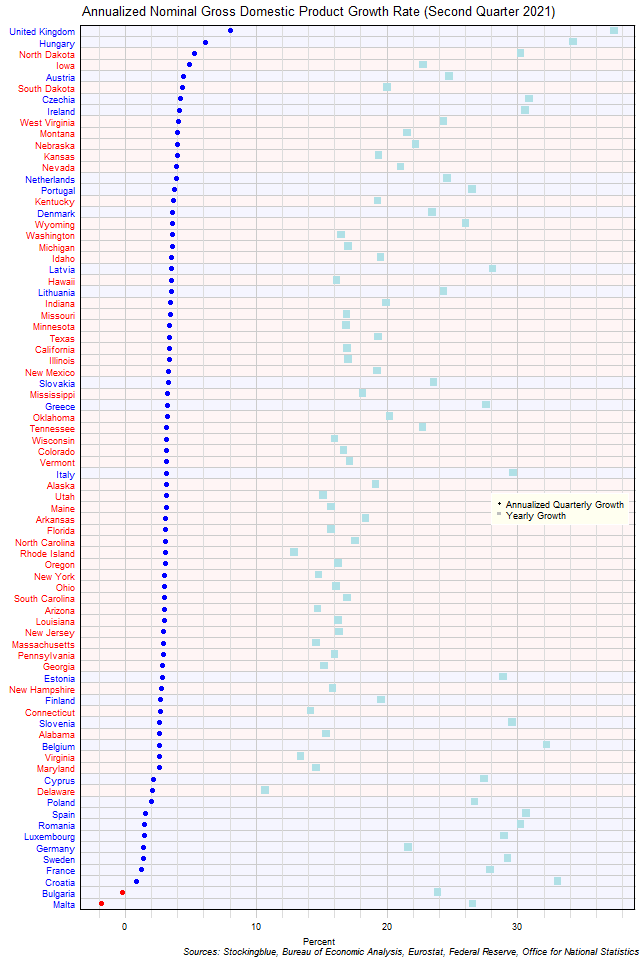The chart above shows the annualized nominal gross domestic product (GDP) quarterly growth rate when the GDP is priced in US dollars in each EU and US state as of the second quarter of 2021 and the growth rate from one year prior.  Bulgaria and Malta were the only states to contract over the previous quarter.

# EU GDP Growth Rate by State, Second Quarter 2021

Nov 23, 2021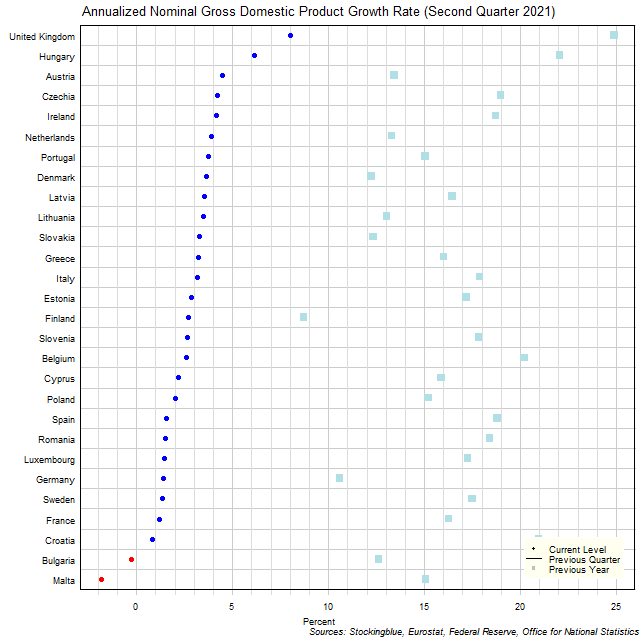The chart above shows the annualized nominal gross domestic product (GDP) quarterly growth rate in each EU state as of the second quarter of 2021 and the growth rate from one year prior.  Two states' economies contracted over the previous quarter.

# US GDP Growth Rate by State, Second Quarter 2021

Nov 22, 2021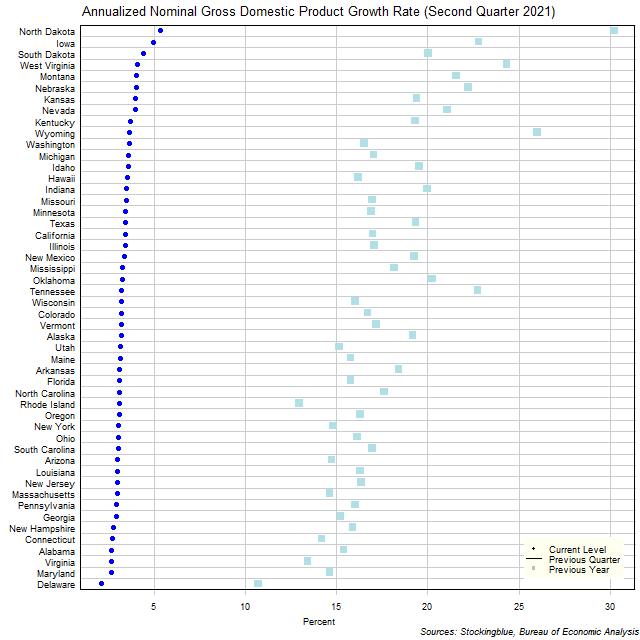The chart above shows the annualized nominal gross domestic product (GDP) quarterly growth rate in each US state as of the second quarter of 2021 and the growth rate from one year prior.  Every single state's economy grew over the previous quarter.

# Per Capita GDP by EU and US Region, Second Quarter 2021

Nov 19, 2021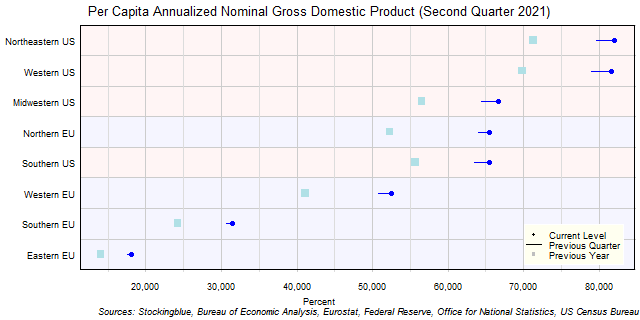The chart above shows the per capita annualized nominal gross domestic product (GDP) in each EU and US region as of the second quarter of 2021 in US dollars, the change from the previous quarter, and the per capita GDP one year prior.  Every single region's economy grew over the past quarter.

# Per Capita GDP by EU Region, Second Quarter 2021

Nov 18, 2021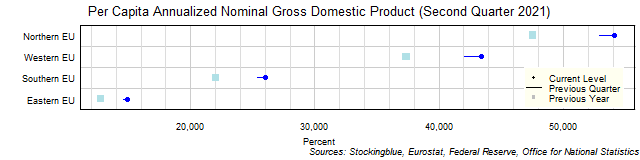The chart above shows the per capita annualized nominal gross domestic product (GDP) in each EU region as of the second quarter of 2021 in euros, the change from the previous quarter, and the per capita GDP one year prior.  All four regions grew over the past quarter and over the past year.

# Per Capita GDP by US Region, Second Quarter 2021

Nov 17, 2021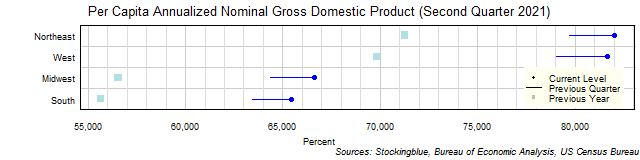The chart above shows the per capita annualized nominal gross domestic product (GDP) in each US region as of the second quarter of 2021 in dollars, the change from the previous quarter, and the per capita GDP one year prior.  Every single region's economy grew over the past quarter and over the past year.

Older